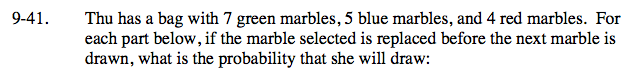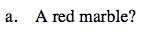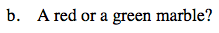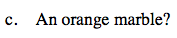Home > ACC7 > Chapter cc29 > Lesson cc29.1.2 > Problem9-41

9-41.
1. Thu has a bag with 7 green marbles, 5 blue marbles, and 4 red marbles. For each part below, if the marble selected is replaced before the next marble is drawn, what is the probability that she will draw. Homework Help ✎

1. A red marble?

2. A red or a green marble?

3. An orange marble?Remember that probability can be written as a fraction:

$\frac{\text{\# of red marbles}} {\text{Total \# of marbles}}$

How many red marbles are there?
How many total marbles?See part (a).

$\frac{11}{16}$See part (a).